12.03.2021 г.

# Interpolation and sampling: why do we need them for projective transformation in image processing?

Today we’ll be discussing the not so obvious moments of a seemingly simple process: correction of projective distortions in an image. Just like we often have to do in everyday life, we had to choose what is more important to us here: quality or speed. In order to reach some kind of balance, we remembered the algorithms that were actively researched in the 80s and 90s as a part of a structural texture rendering and are rarely brought up when it comes to digital image processing these days.

A pinhole camera model which pretty decently models short focal length cameras of mobile phones shows that the images of a flat object at different camera angles are related through a projective transformation. The general form of the projective transformation would be as follows: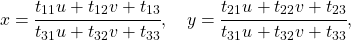where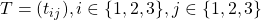is a projective transformation matrix,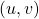and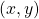are the coordinates in the original and the transformed images.

Geometric transformation of an image

The projective transformation of an image is one of the possible geometric transformations (transformations which imply the transition of the points of an original image transition into the points of the final image in accordance with a certain law).

In order to figure out how to solve the problem of the geometric transformation of a digital image, we need to take into consideration the model of its formation from the optical image on the camera matrix. According to G. Wolberg , our algorithm is supposed to approximate the following process:

1. Recovering the optical image from the digital one.
2. The geometric transformation of the optical image.
3. The transformed image sampling.

The optical image is a function of two variables defined on a continuous set of points. This process is hard to reproduce directly since we will have to set the form of an optical image and process it analytically. The reverse mapping method is often used to facilitate this process:

1. The sampling grid is selected on the plane of a final image — some points that will be used as a reference to estimate the values of the pixels in the final image (it can be the center of each pixel, or a few points per pixel).
2. Using the inverse geometric transformation, this grid is transferred into the plane of the original image.
3. The value is estimated for each sample of the grid. Since a sample will not necessarily be located at a point with integer coordinates, we are going to need to apply some interpolation to the value of the image, for example, interpolation using the adjacent pixels.
4. Now we can estimate the pixel values based on the grid reports.

In this case, the third step is the optical image reconstruction, and the first and the fourth steps correspond to the sampling process.

Interpolation

We will be reviewing only the simplest kinds of interpolation here — those that can be represented as convolutions of an image with an interpolation kernel. In the context of image processing, adaptive interpolation algorithms would be more suitable because they allow maintaining clear object edges in an image, but their computational complexity is significantly higher. And for this reason, we are not interested in them.

We’ll be considering the following interpolation methods:

• Nearest neighbours interpolation,
• Bilinear interpolation,
• Bicubic interpolation,
• Cubic B-spline interpolation,
• Cubic Hermite spline interpolation, using 36 points.

Another important parameter of interpolation is precision. If we assume that the digital image was produced out of the optical image using an approach of point sampling in the center of the pixel and believe that the original image was continuous, then the perfect reconstruction function would be a low frequency filter (with a frequency ½, see the Kotelnikov sampling theorem — https://en.wikipedia.org/wiki/Nyquist%E2%80%93Shannon_sampling_theorem).

Let’s compare the Fourier spectrums of our interpolation kernels with a low frequency filter (the images demonstrate a one-dimensional case).

So what then? Can we just take a kernel with a decent spectrum and get relatively precise results? Well, actually, we can’t because we made two assumptions earlier: we assumed that we have the value of the image pixel and that the image is continuous. While neither one of them is a part of a good model of image generation because the camera matrix sensors are not point sensors, and there is a lot of data in the edges of the object — the discontinuities. That’s why we have to understand that the interpolation result will always be different from the original optical image.

But we still have to solve our problem somehow. We’ll briefly review the pros and cons of each method in practical terms. And the easiest way to do this is to zoom in the image (in this case — 10-fold).

Nearest-neighbor interpolation

It’s the easiest and the fastest kind of interpolation. However, it leads to considerable artifacts.

Bilinear interpolation

It has a superior quality compared to the previous one, but this interpolation is more complex computation-wise and, on top of that, it blurs the edges of the objects in the image.

Bicubic interpolation

It works even better for the continuous areas of an image, but it causes the halo effect at the edges (a darker line along the dark side of the edge, and the lighter line along the light edge). In order to avoid this effect, we will need to use a non-negative convolution kernel. For example, a cubic B-spline.

B-spline interpolation

Due to its very narrow spectrum, there is a significant blur of an image (but it has great noise-reduction properties which can be helpful).

Cubic Hermite spline interpolation

This spline requires estimating the partial derivative values in each pixel of an image. If we choose a two-point difference scheme to approximate, we’ll get the bicubic interpolation kernel. That’s why we want to use a four-point scheme here.

Let’s compare these methods based on the number of memory accesses (the number of pixels in the original image for the interpolation at one point) and the number of multiplications per point.

We can see that the last three methods are much more computationally complex than the first two methods.

Sampling

Here’s the step that unjustly has been paid very little attention to as of late. The simplest way to perform projective transformation of an image is to estimate the value of each pixel for the final image using the value that is a result of the inverse transformation of its center onto the plane of the original image (based on the used interpolation method). This approach is called sampling by pixel. However, in the areas where the image is compressed, this can lead to the significant artifacts as a result of aliasing errors when the sampling frequency is insufficient.

We are going to show compression artifacts using a Russian national passport and one of its text fields — the place of birth field (here — Arhangelsk city (ГОР. АРХАНГЕЛЬСК)) that was compressed using sampling by pixel and the FAST algorithm. We’ll be mentioning this method later and talking about it more.

We can see that the text in the left image became unreadable. And it’s understandable since we only take one point from the whole area of the original image!

Since we were not able to get an estimate by one pixel, why wouldn’t we choose more references for a pixel and approximate the results? This method is called supersampling. It does improve the quality but the computational complexity increases in proportion to the number of pixel references.

The methods that are more computationally effective were developed at the end of the last century when the problem of texture rendering on the flat objects was relevant and was extensively researched. One of these methods is the transformation using the mip-map structure. The mip-map is a pyramid of images consisting of the original image itself and its copies, each of which is a power of two smaller than the previous image. We estimate the typical compression ratio for each pixel, and choose the adequate level of the pyramid according to this ratio as an original image.There are different approaches for estimating the right mip-map level (for more detail see ). Here we’ll be using the method based on the estimate of partial derivatives using the known matrix of the projective transformation. However, in order to avoid the artifacts in those areas of the final image where one level of the mip-map structure transitions into the next one, linear interpolation between two neighboring levels of the pyramid is usually used (it does not significantly increase the computational complexity since the coordinates of the points of the adjacent levels are explicitly connected).

But mip-map method doesn’t account for a fact that image compression might be anisotropic (stretched in some direction). This problem can be partially solved using a rip-map. It’s a structure where the images compressed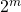times horizontally and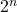times vertically are stored independently. In this case, when we find the horizontal and vertical compression ratio in a given point of the final image, we can do the interpolation of the results from the four copies of the original images that were compressed into the right number of times. But this method is not perfect either, since it doesn’t take into account that the anisotropic direction is different from the directions that are parallel to the edges of the original image.

The FAST (Footprint Area Sampled Texturing) algorithm can partially solve this problem . It combines the ideas of the mip-map method and supersampling. We estimate the compression ratio based on the axis of the lowest anisotropy and choose the number of counts in proportion to the ratio between the smallest axis and the largest one.

Before we start comparing these methods by their computational complexity, we’ll mention that it would be rational to make the following substitution in order to make the calculation of the inverse projective transformation faster: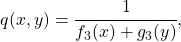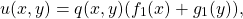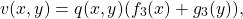where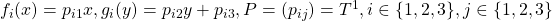, P is the matrix of the inverse projective transformation. Since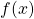and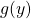are functions of one variable, we can pre-calculate them in time proportional to the linear dimension of the image. Then we are going to need only one division and two multiplications to calculate the coordinates of the pre-image of a pointof the final image. We can apply a similar trick to the partial derivatives that are used to identify the level in the mip-map and rip-map structures.

Now we are ready to compare the results based on their computational complexity.

And here’s the visual comparison (from left to right — samping by pixel, supersampling on 49 counts, mip-map, rip-map, FAST).

The experiment

Now let’s compare our results experimentally. Let’s build a projective transformation algorithm that will combine all 5 interpolation methods and all 5 sampling methods (25 options in total). Let’s take 20 images of the documents cut to the dimensions of 512 by 512 pixels, and generate 10 sets out of 3 projective transformation matrices, so that each set is equivalent to the identity transformation, and let’s compare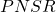of the original image and the transformed one. We’ll remind that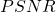is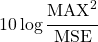, where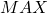is a maximum of the original image, and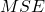is a standard deviation of the final image from the original one. The higher theis, the better. We will measure the operating time of the projective transformation on ODROID-XU4 with the ARM Cortex-A15 processor (2000 MHz and 2GB RAM).

The monstrosity of a table containing the results:

What conclusions can we make about projective transformation in image processing?

• The use of the nearest-neighbor interpolation or sampling by pixel results in the low quality (it was obvious based on the examples we mentioned earlier).
• The Hermite polynomial interpolation on 36 points and supersampling are computationally expensive methods.
• The use of the bicubic interpolation and the B-spline interpolation with any sampling method, except for sampling by pixel, is very time-consuming as well.
• Rip-map’s operating time is comparable to FAST, while its quality is considerably worse and it requires 9 times more additional memory (the choice is obvious here, right?).
• Sampling using mip-map and the B-spline interpolation demonstrated low PSNR because they cause a relatively strong blurring of an image which is not always considered to be a serious issue.

If we want to get the good quality results while the performance speed is not too low, we’d want to consider the bilinear interpolation combined with the sampling that uses mip-map or FAST. However, if we already know that the projective distortion is very weak, we can use the sampling by pixel combined with the bilinear interpolation or even the nearest-neighbor interpolation in order to increase the speed. If we aim for high quality and the operating time is not very limited, we can apply the bicubic or adaptive interpolation paired with a moderate supersampling (for example, the adaptive supersampling that depends on the local compression ratio).

P.S. The publication is prepared based on the paper: A. Trusov and E. Limonova, “The analysis of projective transformation algorithms for image recognition on mobile devices,” ICMV 2019, 11433 ed., Wolfgang Osten, Dmitry Nikolaev, Jianhong Zhou, Ed., SPIE, Jan. 2020, vol. 11433, ISSN 0277-786X, ISBN 978-15-10636-43-9, vol. 11433, 11433 0Y, pp. 1-8, 2020, DOI: 10.1117/12.2559732.

### IDENTITY DOCUMENT SCANNING

Green AI-powered scanner SDK of ID cards, passports, driver’s licenses, residence permits, visas, and other ids, more than 1810+ types in total. Provides eco-friendly, fast and precise scanning SDK for a smartphone, web, desktop or server, works fully autonomously. Extracts data from photos and scans, as well as in the video stream from a smartphone or web camera, is robust to capturing conditions. No data transfer — ID scanning is performed on-device and on-premise.

### CREDIT CARDS, BARCODES, MRZ SCANNING

Automatic scanning of machine-readable zones (MRZ); all types of credit cards: embossed, indent-printed, and flat-printed; barcodes: PDF417, QR code, AZTEC, DataMatrix, and others on the fly by a smartphone’s camera. Provides high-quality MRZ, barcode, and credit card scanning in mobile applications on-device regardless of lighting conditions. Supports card scanning of 21 payment systems.

### DOCUMENT & FORM SCANNING

Automatic data extraction from business and legal documents: KYC/AML questionnaires, applications, tests, etc, administrative papers (accounting documents, corporate reports, business forms, and government forms - financial statements, insurance policies, etc). High-quality Green AI-powered OCR on scans and photographs taken in real conditions. Total security: only on-premise installation. Automatically scans document data in 2 seconds on a modern smartphone.

### COMPUTATIONAL IMAGING AND TOMOGRAPHY

Green AI for Tomographic reconstruction and visualization. Algorithmization of the image reconstruction process directly during the X-ray tomographic scanning process. We aspire to reduce the radiation dose received during the exposure by finding the optimal termination point of scanning.

Send Request